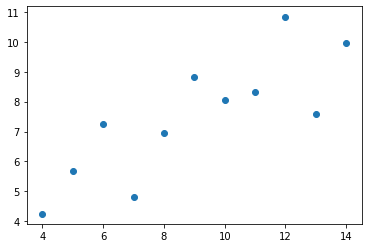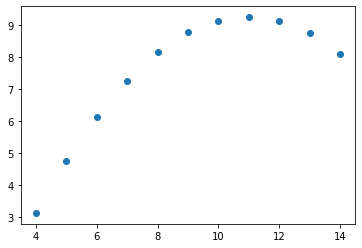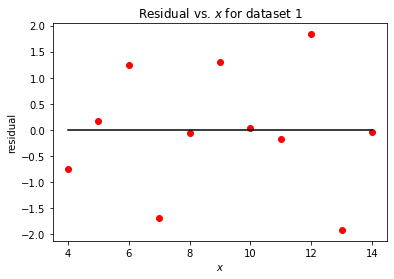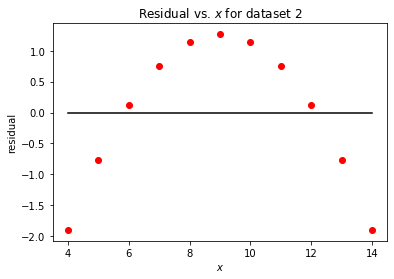# Lecture 13 – Data 100, Summer 2020¶

by Suraj Rampure

In :
import pandas as pd
import numpy as np
import matplotlib.pyplot as plt
import sklearn.linear_model as lm

import plotly.express as px
import plotly.graph_objects as go

import seaborn as sns

In :
nba = pd.read_csv('nba18-19.csv')

In :
nba

Out:
Rk Player Pos Age Tm G GS MP FG FGA ... FT% ORB DRB TRB AST STL BLK TOV PF PTS
0 1 Álex Abrines\abrinal01 SG 25 OKC 31 2 19.0 1.8 5.1 ... 0.923 0.2 1.4 1.5 0.6 0.5 0.2 0.5 1.7 5.3
1 2 Quincy Acy\acyqu01 PF 28 PHO 10 0 12.3 0.4 1.8 ... 0.700 0.3 2.2 2.5 0.8 0.1 0.4 0.4 2.4 1.7
2 3 Jaylen Adams\adamsja01 PG 22 ATL 34 1 12.6 1.1 3.2 ... 0.778 0.3 1.4 1.8 1.9 0.4 0.1 0.8 1.3 3.2
3 4 Steven Adams\adamsst01 C 25 OKC 80 80 33.4 6.0 10.1 ... 0.500 4.9 4.6 9.5 1.6 1.5 1.0 1.7 2.6 13.9
4 5 Bam Adebayo\adebaba01 C 21 MIA 82 28 23.3 3.4 5.9 ... 0.735 2.0 5.3 7.3 2.2 0.9 0.8 1.5 2.5 8.9
... ... ... ... ... ... ... ... ... ... ... ... ... ... ... ... ... ... ... ... ... ...
703 528 Tyler Zeller\zellety01 C 29 MEM 4 1 20.5 4.0 7.0 ... 0.778 2.3 2.3 4.5 0.8 0.3 0.8 1.0 4.0 11.5
704 529 Ante Žižić\zizican01 C 22 CLE 59 25 18.3 3.1 5.6 ... 0.705 1.8 3.6 5.4 0.9 0.2 0.4 1.0 1.9 7.8
705 530 Ivica Zubac\zubaciv01 C 21 TOT 59 37 17.6 3.6 6.4 ... 0.802 1.9 4.2 6.1 1.1 0.2 0.9 1.2 2.3 8.9
706 530 Ivica Zubac\zubaciv01 C 21 LAL 33 12 15.6 3.4 5.8 ... 0.864 1.6 3.3 4.9 0.8 0.1 0.8 1.0 2.2 8.5
707 530 Ivica Zubac\zubaciv01 C 21 LAC 26 25 20.2 3.8 7.2 ... 0.733 2.3 5.3 7.7 1.5 0.4 0.9 1.4 2.5 9.4

708 rows × 30 columns

## Recap from Lecture 12¶

In the last lecture, we used this magical package sklearn to determine the optimal value of $\hat{\theta}$ for a linear model that uses AST and 3PA to predict PTS. (The usage of sklearn will be covered in the next lecture.)

Note: We didn't actually cover this part in the video, but it was in the notebook.

In :
model = lm.LinearRegression(fit_intercept = True)

model.fit(nba[['AST', '3PA']], nba['PTS']);


We then looked at the values of the coefficients:

In :
model.coef_

Out:
array([1.64065507, 1.25758096])
In :
model.intercept_

Out:
2.156347047514835

This meant our model was of the form

$$\text{predicted PTS} = 2.1563 + 1.6407 \cdot \text{AST} + 1.2576 \cdot \text{3PA}$$

## Using np.linalg.inv to solve for optimal $\hat{\theta}$¶

We will now use what we know about the solution to the normal equations to determine $\hat{\theta}$ on our own, by hand. We know that for the ordinary least squares model,

$$\hat{\theta} = (\mathbb{X}^T\mathbb{X})^{-1} \mathbb{X}^T\mathbb{Y}$$
In :
X = nba[['AST', '3PA']]
y = nba['PTS']

In :
X.shape

Out:
(708, 2)

Here, our design matrix $\mathbb{X}$ only has two columns. But, in order to incorporate the intercept term (i.e. $\theta_0$), we need to include a column that contains all 1s. This is referred to as the "intercept" or "bias" column. (sklearn handled this for us, because we set fit_intercept = True. This is actually the default behavior in sklearn.)

In :
X.loc[:, 'bias'] = 1

# This line reorders our columns so that column i corresponds to theta_i.
# By default, newly added columns to go to the end of a DataFrame.
# This is not at all necessary; it just makes our resulting theta vector follow a nice order.
X = X[['bias', 'AST', '3PA']]

/opt/anaconda3/lib/python3.7/site-packages/pandas/core/indexing.py:376: SettingWithCopyWarning:

A value is trying to be set on a copy of a slice from a DataFrame.
Try using .loc[row_indexer,col_indexer] = value instead

See the caveats in the documentation: http://pandas.pydata.org/pandas-docs/stable/user_guide/indexing.html#returning-a-view-versus-a-copy

/opt/anaconda3/lib/python3.7/site-packages/pandas/core/indexing.py:576: SettingWithCopyWarning:

A value is trying to be set on a copy of a slice from a DataFrame.
Try using .loc[row_indexer,col_indexer] = value instead

See the caveats in the documentation: http://pandas.pydata.org/pandas-docs/stable/user_guide/indexing.html#returning-a-view-versus-a-copy


In :
X

Out:
bias AST 3PA
0 1 0.6 4.1
1 1 0.8 1.5
2 1 1.9 2.2
3 1 1.6 0.0
4 1 2.2 0.2
... ... ... ...
703 1 0.8 0.0
704 1 0.9 0.0
705 1 1.1 0.0
706 1 0.8 0.0
707 1 1.5 0.0

708 rows × 3 columns

In :
def least_squares(X, y):
return np.linalg.inv(X.T @ X) @ X.T @ y

In :
theta = least_squares(X, y)

In :
theta

Out:
0    2.156347
1    1.640655
2    1.257581
dtype: float64

Here, theta = 2.156. Since the first column of X is the intercept column, this means that $\theta_0 = 2.1563$. Similarly, we have $\theta_1 = 1.6407$ and $\theta_2 = 1.2576$. These are the exact same coefficients that sklearn found for us!

## Residual plots¶

For the simple linear case, let's revisit Anscombe's quartet.

In :
ans = sns.load_dataset('anscombe')

In :
ans1 = ans[ans['dataset'] == 'I']
ans2 = ans[ans['dataset'] == 'II']


Dataset 1 appears to have a linear trend between $x$ and $y$:

In :
plt.scatter(ans1['x'], ans1['y']);While dataset 2 does not:

In :
plt.scatter(ans2['x'], ans2['y']);Let's fit simple linear regression models to both datasets, and look at residual plots of residual vs. $x$.

In :
slr_ans_1 = lm.LinearRegression()
slr_ans_1.fit(ans1[['x']], ans1['y'])
y_pred_ans_1 = slr_ans_1.predict(ans1[['x']])
residual_ans_1 = ans1['y'] - y_pred_ans_1

In :
plt.scatter(ans1['x'], residual_ans_1, color = 'red');
plt.plot([4, 14], [0, 0], color = 'black')
plt.xlabel(r'$x$')
plt.ylabel('residual');
plt.title(r'Residual vs. $x$ for dataset 1');For dataset 1, it appears that the residuals are generally equally spread out, and that there is no trend. This indicates that our linear model was a good fit here. (It is also true that the "positive" and "negative" residuals cancel out, but this is true even when our fit isn't good.)

In :
slr_ans_2 = lm.LinearRegression()
slr_ans_2.fit(ans2[['x']], ans2['y'])
y_pred_ans_2 = slr_ans_2.predict(ans2[['x']])
residual_ans_2 = ans2['y'] - y_pred_ans_2

In :
plt.scatter(ans2['x'], residual_ans_2, color = 'red');
plt.plot([4, 14], [0, 0], color = 'black')
plt.xlabel(r'$x$')
plt.ylabel('residual');
plt.title(r'Residual vs. $x$ for dataset 2');The fit does not appear to be as good here. While the positive and negative residuals cancel out, there is a clear trend – the underlying data has a quadratic relationship, and the residuals reflect that. We'd likely need to increase the complexity of our model here.

As mentioned above, the residuals in both sum to zero, but this is true of any linear model with an intercept term, as discussed in lecture.

In :
residual_ans_1.sum()

Out:
-1.7763568394002505e-14
In :
residual_ans_2.sum()

Out:
1.021405182655144e-14

For the multiple linear regression case, let's go back to the nba data. Let's once again use AST and 3PA to predict PTS.

In :
nba_model = lm.LinearRegression()
nba_model.fit(nba[['AST', '3PA']], nba['PTS'])
pts_pred = nba_model.predict(nba[['AST', '3PA']])
residual_nba = nba['PTS'] - pts_pred

In :
fig = go.Figure()

data_scatter = go.Scatter(x = pts_pred, y = residual_nba,
mode = 'markers',
marker = dict(color = 'red', size = 8), name = 'residuals vs. fitted values')